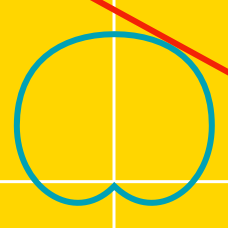Calculus

# Polar Equations - Surface Area

Find the area of the surface formed by revolving the curve $r = 5 \sin\theta$ from $\theta = 0$ to $\theta = \frac{\pi}{2}$ about the $x$-axis.

Find the surface area generated when the curve $r = e^{\theta}$ from $\theta = 0$ to $\theta = \frac{\pi}{2}$ is revolved about the $y$-axis.

Find the area of the surface formed by revolving the curve $r = 5 \sin\theta$ from $\theta = 0$ to $\theta = \frac{\pi}{2}$ about the $y$-axis.

Find the area of the surface formed by revolving the curve $r = 3 \cos(\theta)$ from $\theta = 0$ to $\theta = \frac{\pi}{2}$ about the $x$-axis.

Find the area of the surface formed by revolving the curve $r = 5 \cos{\theta}$ from $\theta = 0$ to $\theta = \frac{\pi}{2}$ about the $y$-axis.

×